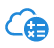##Calculation Tools

The following tools can be used to calculate the characteristics of your device, including: power losses, design values, peripheral circuit constants, and so on. Please note that you must agree to the terms of the Software License Agreement to use the calculation tools. Tap the button with the Part number to open the License Agreement Screen.

##Calculation Tools

The following tools can be used to calculate the characteristics of your device, including: power losses, design values, peripheral circuit constants, and so on. Please note that you must agree to the terms of the Software License Agreement to use the calculation tools.

High Voltage 3-phase Brushless DC Motor Drivers
LED Drivers
DC/DC Converter ICs

### High Voltage 3-phase Brushless DC Motor Drivers

Part Number Parameter Calculation Tool

SCM

SCM1242MF Loss calculation DT0051
SCM1243MF (Not recommended for new design) Loss calculation DT0051
SCM1245MF (Not recommended for new design) Loss calculation DT0051
SCM1246MF (Not recommended for new design) Loss calculation DT0051
SCM1256MF Loss calculation DT0051
SCM1261MF Loss calculation DT0051
SCM1263MF Loss calculation DT0051
SCM1265MF Loss calculation DT0051
SCM1272MF Loss calculation DT0051
SCM1274MF Loss calculation DT0051
SCM1276MF Loss calculation DT0051
SCM2007MKF Loss calculation DT0051
SCM2008MKF Loss calculation DT0051

SIM

SIM2601M (Preview) Loss calculation DT0050
SIM2602M Loss calculation DT0052
SIM6811M Loss calculation DT0050
SIM6812M Loss calculation DT0050
SIM6813M (Not recommended for new design) Loss calculation DT0050
SIM6822M (Not recommended for new design) Loss calculation DT0052
SIM6822MV Loss calculation DT0052
SIM6827M (Not recommended for new design) Loss calculation DT0052
SIM6827MV (Preview) Loss calculation DT0052
SIM6880M Loss calculation DT0052

SLA

SLA6805MH Loss calculation DT0052
SLA6846MH Loss calculation DT0052
SLA6868MH Loss calculation DT0050
SLA6870MH Loss calculation DT0050

SMA

SMA6860MH Loss calculation DT0050
SMA6862MH Loss calculation DT0050
SMA6863MH Loss calculation DT0050
SMA6865MH Loss calculation DT0050

SX

SX1A5201E1S (Not recommended for new design) Loss calculation DT0050
SX68001MH Loss calculation DT0050
SX68002MH (Obsolete) Loss calculation DT0050
SX68003MH Loss calculation DT0050
SX68126M Loss calculation DT0050
SX68127M (Preview) Loss calculation DT0050
SX68128M (Preview) Loss calculation DT0050
SX68201M Loss calculation DT0050
SX68203M Loss calculation DT0050
SX68205M Loss calculation DT0050

### LED Drivers

Part Number Parameter Calculation Tool
LC5910S Peripheral Circuit Constants, Design Values, Power Losses DT0024

### DC/DC Converter ICs

Part Number Parameter Calculation Tool
NR263S Peripheral Circuit Constants, Design Values, Power Losses DT0022
NR421A Peripheral Circuit Constants, Design Values, Power Losses DT0023